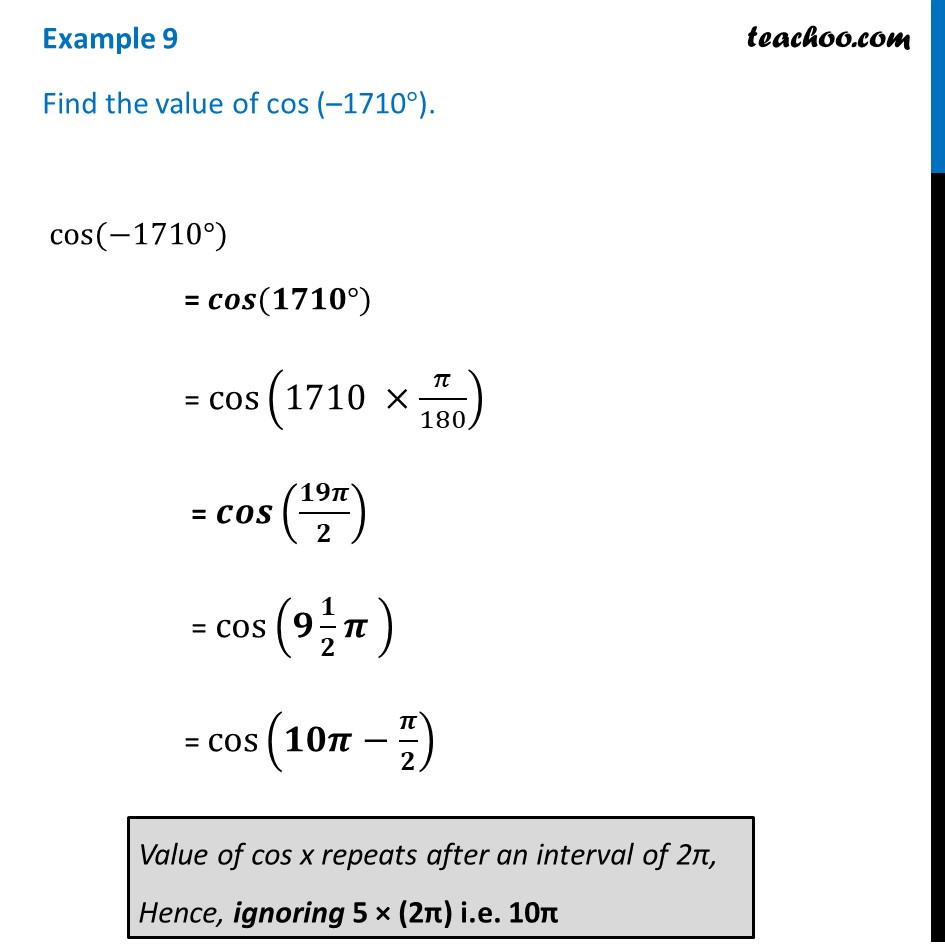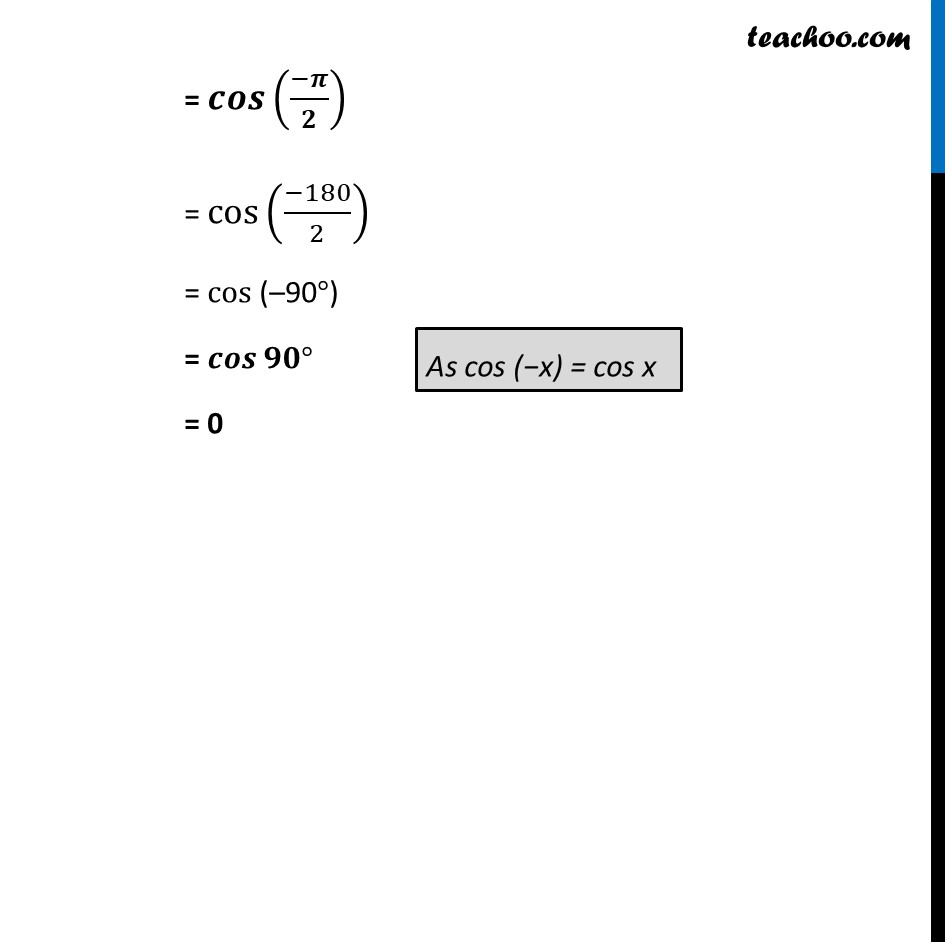Examples

Chapter 3 Class 11 Trigonometric Functions
Serial order wiseLearn in your speed, with individual attention - Teachoo Maths 1-on-1 Class

### Transcript

Example 9 Find the value of cos (–1710°). cos⁡(−1710°) = 𝒄𝒐𝒔⁡(𝟏𝟕𝟏𝟎°) = cos⁡(1710 ×𝜋/180) = 𝒄𝒐𝒔⁡(𝟏𝟗𝝅/𝟐) = cos⁡(𝟗 𝟏/𝟐 𝝅 ) = cos⁡(𝟏𝟎𝝅−𝝅/𝟐) = 𝒄𝒐𝒔⁡((−𝝅)/𝟐) = cos⁡((−180)/2) = cos⁡(–90°) = 𝒄𝒐𝒔⁡𝟗𝟎° = 0